# AIIMS Chemistry Mock Test - 3

## 60 Questions MCQ Test AIIMS Mock Tests & Previous Year Papers | AIIMS Chemistry Mock Test - 3

Description
Attempt AIIMS Chemistry Mock Test - 3 | 60 questions in 60 minutes | Mock test for NEET preparation | Free important questions MCQ to study AIIMS Mock Tests & Previous Year Papers for NEET Exam | Download free PDF with solutions
QUESTION: 1

Solution:
QUESTION: 2

Solution:
QUESTION: 3

### Water gas is a mixture of

Solution:
QUESTION: 4

Amongst the following compounds which can be most easily sulphonated is

Solution:
QUESTION: 5

Which of the following contains three pairs of electrons in the valence shell?

Solution:
QUESTION: 6

The most reactive among the following towards sulphonation is

Solution:
QUESTION: 7

Benzene reacts with CH₃COCl in the presence of AlCl₃ to give

Solution:
QUESTION: 8

Freon is used as a

Solution:
QUESTION: 9

In which of the following pairs, both molecules possess dipole moment?

Solution:
QUESTION: 10

H2 dissociates in the presence of light only when Hg vapours are present. It is an example of

Solution:
QUESTION: 11

Which of the following gas molecule has the maximum specific heat at constant pressure?

Solution:
QUESTION: 12

In the following question, a Statement of Assertion (A) is given followed by a corresponding Reason (R) just below it. Read the Statements carefully and mark the correct answer-
Assertion(A): In a dry cell zinc acts as cathode and carbon rod as anode.
Reason(R): A dry cell has a potential of nearly 1.0 V.

Solution:
QUESTION: 13

In the following question, a Statement of Assertion (A) is given followed by a corresponding Reason (R) just below it. Read the Statements carefully and mark the correct answer-
Assertion(A): Iron is coated with zinc in galvanisation process.
Reason(R): Zinc works as cathodic protection against rusting of iron.

Solution:
QUESTION: 14

In the following question, a Statement of Assertion (A) is given followed by a corresponding Reason (R) just below it. Read the Statements carefully and mark the correct answer-
Assertion(A): Respiration is a chemical change which is the opposite of photosynthesis.
Reason(R): During respiration glucose is synthesised in our system .

Solution:
QUESTION: 15

Rate of formation of SO3 in the following reaction
2SO2 + O2 → 2SO3
is 100g min−1, Hence, rate of disappearance of O2 is:

Solution: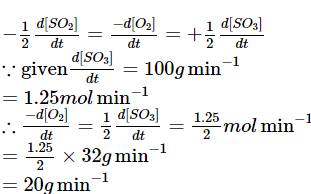QUESTION: 16

In the following question, a Statement of Assertion (A) is given followed by a corresponding Reason (R) just below it. Read the Statements carefully and mark the correct answer-
Assertion(A): Cuprous ion is colourless while cupric ion is blue in aqueous solution.
Reason(R): Cuprous ion has unpaired electron while cupric ion don't.

Solution:
QUESTION: 17

In the following question, a Statement of Assertion (A) is given followed by a corresponding Reason (R) just below it. Read the Statements carefully and mark the correct answer-
Assertion(A): When CoCl3.5NH3 is dissolved in water and treated with excess AgNO3 solution , it gives only two moles of AgCl.
Reason(R): Third chloro is hydrogen bonded to NH3.

Solution:
QUESTION: 18

In the following question, a Statement of Assertion (A) is given followed by a corresponding Reason (R) just below it. Read the Statements carefully and mark the correct answer-
Assertion(A): All F-S-F angle in SF4 is less than 180 degrees.
Reason(R): The lone pair-bond pair repulsion is weaker than bond pair-bond pair repulsion.

Solution:
QUESTION: 19

In the following question, a Statement of Assertion (A) is given followed by a corresponding Reason (R) just below it. Read the Statements carefully and mark the correct answer-
Assertion(A): Boiling points of cis isomers are higher than trans-isomers.
Reason(R): Dipole moments of cis isomers are higher than trans isomers.

Solution:
QUESTION: 20

In the following question, a Statement of Assertion (A) is given followed by a corresponding Reason (R) just below it. Read the Statements carefully and mark the correct answer-
Assertion(A): R-C ≡ O+ is more stable than R-C+ = O.
Reason(R): Resonance in carbonyl compound provides C+ and O-.

Solution:
QUESTION: 21

In the following question, a Statement of Assertion (A) is given followed by a corresponding Reason (R) just below it. Read the Statements carefully and mark the correct answer-
Assertion(A): In titration of strong acid and strong base, we can use phenol-phthalein as indicator.
Reason(R): At the end point pH of solution is in between 8-10.

Solution:
QUESTION: 22

In the following question, a Statement of Assertion (A) is given followed by a corresponding Reason (R) just below it. Read the Statements carefully and mark the correct answer-
Assertion(A): Carboxylic acids do nt give characteristic reactions of the carbonyl group.

Reason (R): The carbonyl carbon of carboxylic acid is involved in resonance.

Solution:
QUESTION: 23

In the following question, a Statement of Assertion (A) is given followed by a corresponding Reason (R) just below it. Read the Statements carefully and mark the correct answer-
Assertion(A): [Pt(NH2)2Cl2] is tetrahedral.
Reason(R): The oxidation state of platinum is +2.

Solution:
QUESTION: 24

In the following question, a Statement of Assertion (A) is given followed by a corresponding Reason (R) just below it. Read the Statements carefully and mark the correct answer-
Assertion(A): Industrial method of preparation of H₂O₂ involves 2-ethylanthra quinol.
Reason(R): Process becomes cyclic.

Solution:
QUESTION: 25

In the following question, a Statement of Assertion (A) is given followed by a corresponding Reason (R) just below it. Read the Statements carefully and mark the correct answer-
Assertion(A): The overall reactivity of acid derivative follows the order:
Acetyl Chloride > Acid Anhyaride > Acid ester > Acid Amide.
Reason(R): Reactivity order as per the basicity order of leaving group Cl- > RCOO- > RO - > NH2-.

Solution:
QUESTION: 26

In the following question, a Statement of Assertion (A) is given followed by a corresponding Reason (R) just below it. Read the Statements carefully and mark the correct answer-
Assertion(A): All enzymes are proteins but all proteins are not enzymes.
Reason(R): Keratin is an enzymes.

Solution:

Keratin refers to a family of fibrous structural proteins. Keratin is the key structural material making up the outer layer of human skin, hair and nails.

QUESTION: 27

In the following question, a Statement of Assertion (A) is given followed by a corresponding Reason (R) just below it. Read the Statements carefully and mark the correct answer-
Assertion(A): Narcotics are opium derivatives.
Reason(R): Narcotic is an analgesic.

Solution:
QUESTION: 28

In the following question, a Statement of Assertion (A) is given followed by a corresponding Reason (R) just below it. Read the Statements carefully and mark the correct answer-
Assertion(A): Conc. H2SO4 reacts with KCl to give Cl2 gas.
Reason(R): HCl cannot be oxidising by H2SO4.

Solution:
QUESTION: 29

In the following question, a Statement of Assertion (A) is given followed by a corresponding Reason (R) just below it. Read the Statements carefully and mark the correct answer-
Assertion(A): Ethanol is liquid but dimethyl ether is a gas.
Reason(R): In ethanol, carbon as well as oxygen are sp3 hybridised.

Solution:
QUESTION: 30

In the following question, a Statement of Assertion (A) is given followed by a corresponding Reason (R) just below it. Read the Statements carefully and mark the correct answer-
Assertion(A): The mobility of sodium ion is lower than that of potassium ion.
Reason(R): The ionic mobilities depend upon the effective radius of the ion.

Solution:
QUESTION: 31

In the following question, a Statement of Assertion (A) is given followed by a corresponding Reason (R) just below it. Read the Statements carefully and mark the correct answer-
Assertion(A): For a monatomic gas Poisson's ratio is equal to 1.66, whereas for diatomic gas it is equal to 1.4.
Reason(R): The ratio of molar heat capacities of a gas is called Poisson's ratio.

Solution:
QUESTION: 32

In the following question, a Statement of Assertion (A) is given followed by a corresponding Reason (R) just below it. Read the Statements carefully and mark the correct answer-
Assertion(A): [Ti(H2O)6]3+ is coloured while [Sc(H2O)6]3+ is colourless.
Reason(R): d-d transition is not possible in[Sc(H2O)6]3+.

Solution:
QUESTION: 33

Reaction that takes place at graphite anode in dry cell is

Solution:
QUESTION: 34

The rate of diffusion of hydrogen gas is

Solution:
QUESTION: 35

On passing 0.1 faraday of electricity through fused sodium chloride, the amount of chloride liberated is (At. mass of Cl = 35.45)

Solution:
QUESTION: 36

Which one of the following has highest electropositive character?

Solution:
QUESTION: 37

A solution of sodium metal in liquid ammonia is strongly reducing due to the presence of

Solution:
QUESTION: 38

Which of the following statments is false for alkali metals?

Solution:
QUESTION: 39

Which of the following acid is weakest?

Solution:
QUESTION: 40

Which of the following alkaline earth metal is most abundant in the earth's crust?

Solution:
QUESTION: 41

Hydrogen peroxide is used as

Solution:
QUESTION: 42

The pH of solution formed by mixing 40 ml of 0.10 M HCl with 10 ml of 0.45 M of NaOH is

Solution:
QUESTION: 43

The indicator used in the titration of sodium carbonate with sulphuric acid is

Solution:
QUESTION: 44

The Ksp of CuS,Ag₂S and HgS are 10⁻31, 10⁻44, 10⁻54 respectively.
The solubility of these sulphides are in the order

Solution:
QUESTION: 45

In which of the following acid-base titration, pH is greater at eqivalence point?

Solution:
QUESTION: 46

Which of the following reagents is used to distinguish acetone and acetophenone?

Solution:
QUESTION: 47

Among the following compounds, which will react with acetone to give a product containing > C ═ N-?

Solution:
QUESTION: 48

Solution:
QUESTION: 49

Arrange in order of decreasing trend towards ES (electrophilic substitution) reactions: Chlorobenzene (I), Benzene (II), Anilinium chloride (III), Toluene (IV)

Solution:
QUESTION: 50

Select correct statements(s):

Solution:
QUESTION: 51

Identify the correct order of reactivity in electrophilic substitution action of the following compounds.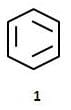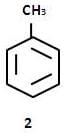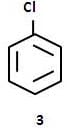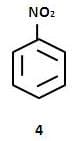Solution:
QUESTION: 52

To obtain pure silicon, SiH₄ is heated at

Solution:
QUESTION: 53

Which type of glass has a property to cut off ultra-violet rays?

Solution:
QUESTION: 54

The number of ions in a unit structure of NaCl are

Solution:
QUESTION: 55

Azeotropic mixture of HCl and water has

Solution:
QUESTION: 56

The mole fraction of the solute in one molal aqueous solution is

Solution:
QUESTION: 57

The formula weigh of H₂SO₄ is 98. The weight of the acid in 400 ml of 0.1 M solution is

Solution:
QUESTION: 58

Which of the following would not have a trans isomer?

Solution:
QUESTION: 59

The amount of Zn required to produce 224 ml of H2 at STP on treatment with dil H2SO4 will be

Solution:
QUESTION: 60

Bell metal is an alloy of

Solution:Use Code STAYHOME200 and get INR 200 additional OFF Use Coupon Code

### How to Prepare for NEET

Read our guide to prepare for NEET which is created by Toppers & the best Teachers